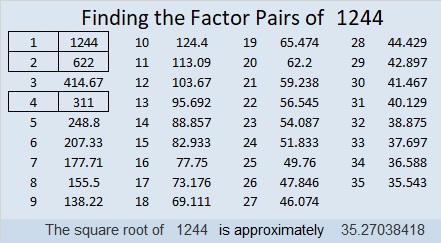# 1244 and Level 2

If you can find the common factors of the clues in each row or column of this puzzle, then you can solve this puzzle. Be sure to only write numbers from 1 to 10 as those factors, and I’m sure you can succeed.Print the puzzles or type the solution in this excel file: 10-factors-1242-1250

Here are some facts about the number 1244:

• 1244 is a composite number.
• Prime factorization: 1244 = 2 × 2 × 311, which can be written 1244 = 2² × 311
• The exponents in the prime factorization are 2 and 1. Adding one to each and multiplying we get (2 + 1)(1 + 1) = 3 × 2  = 6. Therefore 1244 has exactly 6 factors.
• Factors of 1244: 1, 2, 4, 311, 622, 1244
• Factor pairs: 1244 = 1 × 1244, 2 × 622, or 4 × 311
• Taking the factor pair with the largest square number factor, we get √1244 = (√4)(√311) = 2√311 ≈ 35.270381244 is the sum of the cubes of the first four triangular numbers:
1³ + 3³ + 6³ + 10³ =1244

1244 is a palindrome in a couple of different bases:
It’s 878 in BASE 12 and
282 in BASE 23